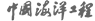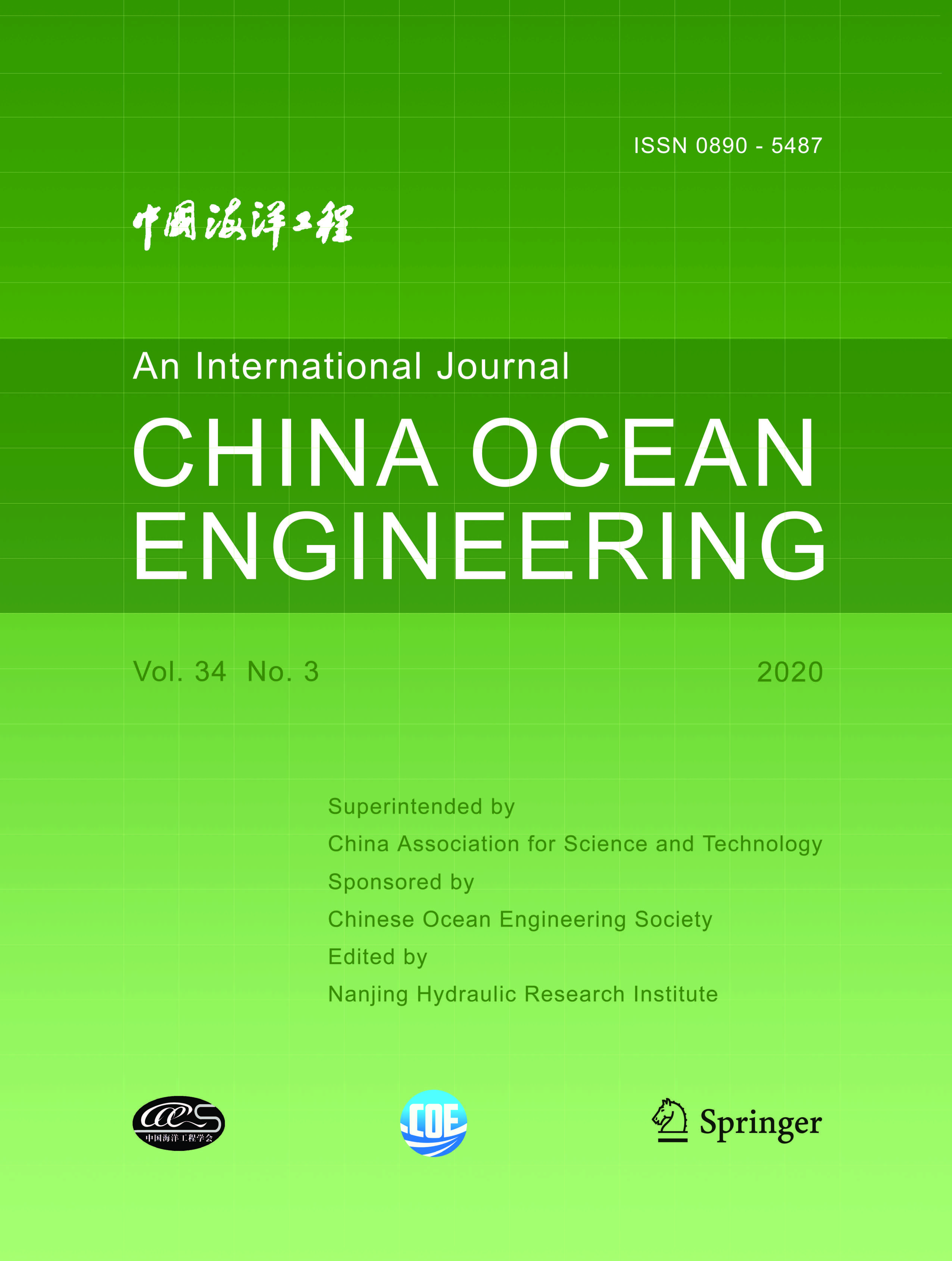ISSN  0890-5487 CN 32-1441/P

## 1999 Vol.(4)

Display Mode：          |

1999, (4): -.
[Abstract](375)
Abstract:
To determine the optimal length of the cross-bay bridge at the Tieshan Port and the impact of the bridge on the hydrological environment, a 2-D numerical model is adopted to calculate the variation of tidal level, tidal current field and tidal discharge caused by different lengths of the bridge.
1999, (4): -.
[Abstract](331)
Abstract:
1999, (4): -.
[Abstract](330)
Abstract:
To deal with the problems concerning the shore boundary, moving boundary and engineering boundary which are encountered frequently in 2D tidal current simulation by the finite difference method, the concept of line boundary is introduced and studied here, and then the line boundary technique in common use is proposed in this paper. Analysis of some calculation cases shows that this technique is practical, effective, and simple in 2D tidal current simulation involving different boundaries.
1999, (4): -.
[Abstract](337)
Abstract:
Theoretical studies so far on random wave groups have all been in linear ways. Methods to simulate random wave groups, an important subject in ocean engineering, also employ relationship resulting from a Gaussian process. Many filed measurements have shown that the real sea surface displacement deviates somewhat from Gaussian distribution. Tayfun et al. have further depicted in theory that the envelope spectral peak frequency is constantly zero for a Gaussian process which means that the groupiness factors will be constants, too. In this paper, the effect of nonlinearity on groupiness of a random wave field is examined via the theoretical results derived by Tayfun et al. from an expression of amplitude-modulated Stokes waves. When the surface displacement is treated as a non-Gaussian process, it is found that the group height factors GF1 and GF2 proposed by Zhao et al. and Yu et al. , respectively, depend on a nonlinearity factor as well as a spectrum-bandwidth factor, deferring from the case of a Gaussion process. Comparison between the theoretical results and the field data shows a favorable agreement in consideration of errors from instrumentation and measuring means. The significance of the results is also discussed.
1999, (4): -.
[Abstract](336)
Abstract:
The interaction of water waves with multiple circular cylinders is analysed briefly in this paper. The formula obtained by Linton and Evans is improved to introduce a relation of phase between cylinders. The condition for the existence of the solution has been proved. The numerical results are compared with analytic solutions (Linton and Evans), numerical solutions and experimental data (Isaacson), and good agreement has been found.
1999, (4): -.
[Abstract](328)
Abstract:
1999, (4): -.
[Abstract](351)
Abstract:
Nelson pointed out that the wave breaking criterion (H/d)b for gentle slopes (i < 1/100) , in which H is the wave height and d is the water depth at the breaking point, is smaller than that for beach slopes of i > 1 /100 , i. e., the value of (H/ d) b for gentle slopes may be smaller than 0.6. Goda indicated that the wave breaking criterion given by himself is a result based on theoretical study, so it should be also correct and can be used for gentle beaches, i. e., the value of (H/d)b for gentle slopes may be still larger than 0.7. By use of high order nonlinear wave theory, this problem is analyzed in this study and the reason why there is a large difference between different studies is explained. Moreover, the bottom energy loss during wave propagation is considered and the critical slope for the absence of wave breaking is also analyzed.
1999, (4): -.
[Abstract](327)
Abstract:
A simple probabilistic model for predicting crack growth behavior under random loading is presented. In the model, the parameters c and m in the Paris-Erdogan Equation are taken as random variables, and their stochastic characteristic values are obtained through fatigue crack propagation tests on an offshore structural steel under constant amplitude loading. Furthermore, by using the Monte Carlo simulation technique, the fatigue crack propagation life to reach a given crack length is predicted. The tests are conducted to verify the applicability of the theoretical prediction of the fatigue crack propagation.
1999, (4): -.
[Abstract](341)
Abstract:
Based on the refraction-diffraction theory of irregular waves in the waters of slowly-varying currents and depths, and the generation dissipation theory of wind wave, a model for nonlinear irregular waves in coastal area is developed. In light of the specific conditions of coastal wave character and engineering application , a practical mathematical model for the nonlinear irregular waves is presented, with directional spectrum in coastal area. Coast effect, refraction, whitecapping, bottom friction, current, wind and nonlinear action are considered in this model. The numerical methods and schemes for wave refraction ray, energy conservation of propagation, energy balance of the generation and dissipation of wind waves have been studied. Finally, the model is used for the directional wave spectrum computation in the Daya Bay. Compared with the measured data with 956 wave bouys in the Daya Bay, the model results are in good agreement with the measured results.
1999, (4): -.
[Abstract](341)
Abstract:
The volume of fluid (VOF) method is used to set up a wave flume with an absorbing wave maker of cnoidal waves. Based on the transfer function between wave surface and paddle velocity obtained by the shallow water wave theory, the velocity boundary condition of an absorbing wave maker is introduced to absorb reflected waves that reach the numerical wave maker. For H/d ranging from 0.1 to 0.59 and T g/d from 7.9 to 18.3, the parametric studies have been carried out and compared with experiments.
1999, (4): -.
[Abstract](338)
Abstract:
The propagation, shoaling and breaking of solitary waves on mild slopes are simulated by boundary element method. In this paper, the criterion of breaking solitary waves on mild slopes is discussed. The criterion is that the ratio of horizontal velocity of water particles on the wave crest to wave celerity equals one. However, the case that the ratio of horizontal velocity of water particles on the wave crest to wave celerity is below one but the front face of wave profile becomes vertical is also considered as a breaking criterion. According to the above criteria, the breaking index for slopes 1:10 to 1:25 is studied. The result is compared to other researchers' . The deformation of solitary waves on slopes is discussed and the distribution of fluid velocities at breaking is shown.
1999, (4): -.
[Abstract](325)
Abstract:
1999, (4): -.
[Abstract](354)
Abstract:
1999, (4): -.
[Abstract](324)
Abstract:
1999, (4): -.
[Abstract](319)
Abstract:
This paper presents a refined parabolic approximation model of the mild slope equation to simulate the combination of water wave refraction and diffraction in the large coastal region. The bottom friction and weakly nonlinear term are included in the model. The difference equation is established with the Crank-Nicolson scheme. The numerical test shows that some numerical prediction results will be inaccurate in complicated topography without considering weak nonlinearity; the bottom friction will make wave height damping and it can not be neglected for calculation of wave field in large areas.

### Current Issue1. Editor-in-Chief:

2. Superintended by:

CHINA ASSOCIATION FOR SCIENCE AND TECHNOLOGY

Chinese Ocean Engineering Society （COES）

4. Edited by:

Nanjing Hydraulic Research InstituteAddress: 34 Hujuguan, Nanjing 210024, China Pos: 210024 Tel: 025-85829388 E-mail: coe@nhri.cn

Support by Beijing Renhe Information Technology Co. Ltd E-mail: info@rhhz.net